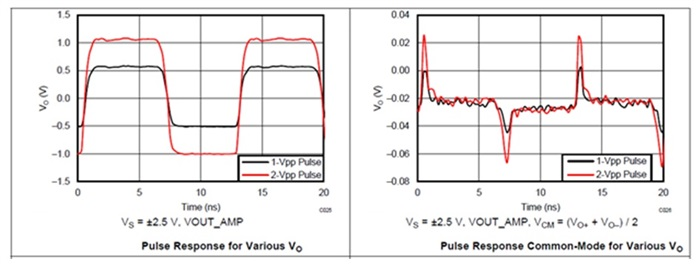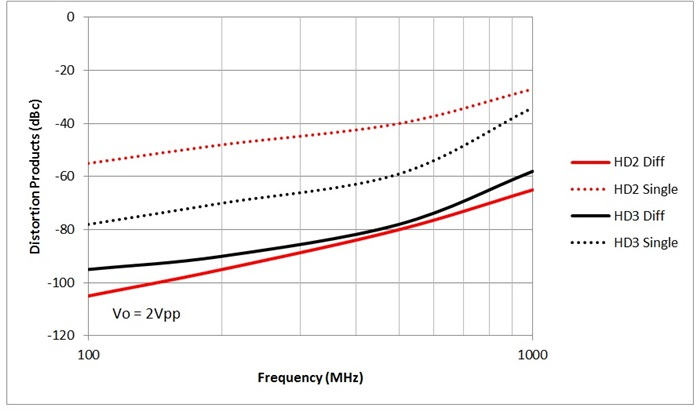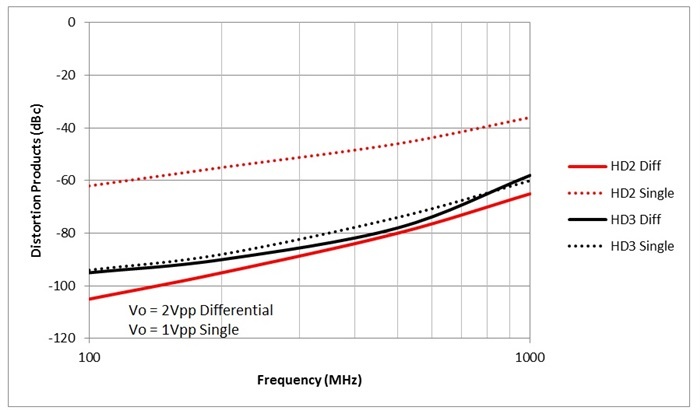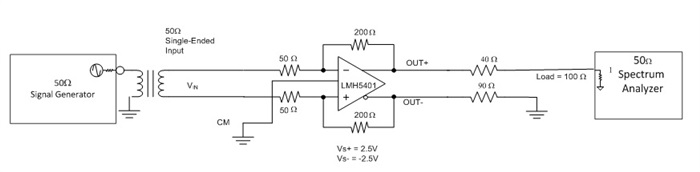# Differential to single ended: What happens when you use only one differential amplifier output

Many applications require the conversion of a differential signal to single ended. Some common examples are an RF DAC buffer or a coaxial cable driver.  Most of the time you can accomplish this with a magnetic transformer, but sometimes a transformer won’t work. If that’s the case, can you use a fully differential amplifier (FDA)? The answer is a definite maybe.

As a refresher, an FDA has two distinct outputs available. The first output is the most commonly used: it consists of the difference between the outputs, . The other output is generally considered a parasitic output; it is the average value of the two outputs . The common-mode output DC level is important; however, its derivative should be zero, meaning that it should have no AC component. This, in fact, will not be the case.

Let’s look at an example scenario. The LMH5401 is an FDA with extraordinarily high bandwidth. The pulse response is shown in Figure 1. At left is the differential output response, and at right is the common-mode response.Figure 1: LMH5401 pulse response, differential (left) and common mode (right)

Now that we have reviewed the two main output modes of an FDA, let’s look at two potential alternative outputs: Out+ alone and Out- alone. For now, let’s use the identity of  and look at what happens to the two primary responses with respect to one output only. For an FDA, the closed-loop gain = ) ; given the same loop gain using only one output, the closed-loop gain  .This makes it clear that using only one amplifier output cuts the gain by 6dB, or by a factor of two. With an amplifier like the LMH5401, you can mitigate this drawback by using different external resistors to set the amplifier gain higher by a factor of two.  Similarly, if you are using FDAs as attenuators, this gain reduction is an added benefit.

Using the same method, the amplifier common mode would change from to  . This conversion shows that when using the single-ended method, the amplifier output common mode is no longer a meaningful concept since the common mode is simply equal to the output

To gain more insight into the amplifier performance, we’ll need to use more sensitive equipment than that used for Figure 1. With a spectrum analyzer, we can measure the amplifier distortion under single-tone conditions with a very high degree of precision. Figure 2 shows distortion measurements for the LMH5401.Figure 2: Distortion measured with differential and single-ended outputs

Figure 2 clearly shows that the single-ended output does not provide the linearity of a differential condition. The output voltage for both conditions is 2Vpp. Note that in the single-ended output condition, one output running 2Vpp is the “same” as a 4Vpp condition for a differential output.

Given this serious handicap of single-ended operation, what happens when we drop the signal amplitude to make the conditions more comparable? Figure 3 shows what happens when you decrease the signal amplitude so that each output is swinging the same voltage, whether the results are measured in single ended or differential mode.Figure 3: Distortion measured with differential and single-ended outputs

Figure 3 clearly shows that the third-order distortion products (HD3) are very similar for a single-ended output or a differential output – as long as you account for the amplitude penalty of single-ended outputs. The results for the second-order distortion products (HD2) are not comparable, however. This is the primary disadvantage of using an FDA with only a single output. While the HD2 of each output will cancel when the outputs are combined into a differential signal, this does not happen with a single-ended output.Figure 4: Test schematic

In conclusion, using a single output from an FDA may work in some limited applications: when the signal is at the lower end of the amplifier’s working frequency range, when the signal amplitudes are small, and when second-order distortion products are not a primary performance metric.

Related technical resources: